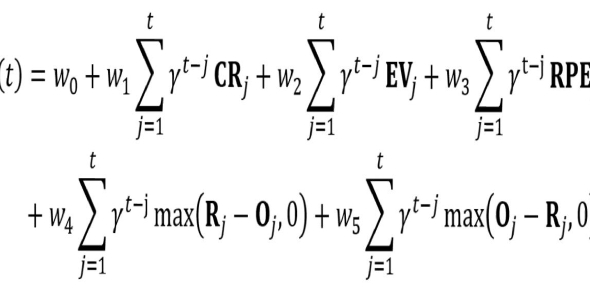# Easy - General Math Equations

Approved & Edited by ProProfs Editorial Team
At ProProfs Quizzes, our dedicated in-house team of experts takes pride in their work. With a sharp eye for detail, they meticulously review each quiz. This ensures that every quiz, taken by over 100 million users, meets our standards of accuracy, clarity, and engagement.
| Written by Tinkerswo
T
Tinkerswo
Community Contributor
Quizzes Created: 1 | Total Attempts: 111
Questions: 17 | Attempts: 111SettingsThis quiz is the easiest level out of the three. The quiz includes addition, multiplication, subtraction and division.

• 1.

### 548 + 296

• A.

844

• B.

956

• C.

563

• D.

845

A. 844
Explanation
The correct answer is 844 because when you add 548 and 296 together, you get a sum of 844.

Rate this question:

• 2.

### 220 + 1006

• A.

5986

• B.

1226

• C.

1500

• D.

3569

B. 1226
Explanation
The correct answer is 1226 because when you add 220 and 1006 together, you get a sum of 1226.

Rate this question:

• 3.

### 682 + 96

• A.

869

• B.

1559

• C.

654

• D.

778

D. 778
Explanation
The correct answer is 778 because when you add 682 and 96 together, you get a sum of 778.

Rate this question:

• 4.

### 45 + 66320

• A.

4589

• B.

66389

• C.

66365

• D.

65932

C. 66365
Explanation
The correct answer is 66365 because when you add 45 and 66320, you get a sum of 66365.

Rate this question:

• 5.

### 3 x 7

• A.

98

• B.

21

• C.

36

• D.

25

B. 21
Explanation
The correct answer is 21 because when you multiply 3 by 7, the result is 21.

Rate this question:

• 6.

### 6 x 5

• A.

30

• B.

21

• C.

40

• D.

36

A. 30
Explanation
The correct answer is 30 because when you multiply 6 by 5, you get the product of 30.

Rate this question:

• 7.

### 8 x 5

• A.

59

• B.

40

• C.

36

• D.

20

B. 40
Explanation
The correct answer is 40 because when you multiply 8 by 5, you get the product of 40.

Rate this question:

• 8.

• A.

25

• B.

65

• C.

58

• D.

36

D. 36
• 9.

### 705 - 392

• A.

698

• B.

626

• C.

613

• D.

589

C. 613
Explanation
The correct answer is 613 because when you subtract 392 from 705, you get 313. However, none of the given options match this result. Therefore, it is likely that there is an error in the question or the options provided. Without further information, it is difficult to determine the correct answer.

Rate this question:

• 10.

### 6978 - 5462

• A.

1516

• B.

1626

• C.

1856

• D.

1511

A. 1516
Explanation
The correct answer is 1516. To find the difference between 6978 and 5462, we subtract the smaller number from the larger number. In this case, 5462 is subtracted from 6978, resulting in 1516.

Rate this question:

• 11.

### 896 - 66

• A.

865

• B.

790

• C.

830

• D.

833

C. 830
Explanation
The correct answer is 830 because when you subtract 66 from 896, you get 830.

Rate this question:

• 12.

### 80 - 9

• A.

91

• B.

78

• C.

71

• D.

85

C. 71
Explanation
The correct answer is 71 because when we subtract 9 from 80, we get 71.

Rate this question:

• 13.

### 1000 - 966

• A.

54

• B.

34

• C.

26

• D.

36

B. 34
Explanation
The given question is asking for the result of subtracting 966 from 1000. When we subtract 966 from 1000, we get 34. Therefore, the correct answer is 34.

Rate this question:

• 14.

### 56 ÷ 7

• A.

9

• B.

4

• C.

6

• D.

8

D. 8
Explanation
The correct answer is 8 because when you divide 56 by 7, you get 8 as the quotient.

Rate this question:

• 15.

### 10 ÷ 2

• A.

8

• B.

10

• C.

5

• D.

6

C. 5
Explanation
When we divide 10 by 2, we get the quotient of 5. This means that if we divide 10 into 2 equal parts, each part will be equal to 5. Therefore, the correct answer is 5.

Rate this question:

• 16.

### 108 ÷ 9

• A.

12

• B.

15

• C.

20

• D.

10

A. 12
Explanation
The correct answer is 12 because when you divide 108 by 9, you are essentially asking how many times 9 can go into 108. Since 9 times 12 equals 108, the answer is 12.

Rate this question:

• 17.

### 45 ÷ 5

• A.

6

• B.

10

• C.

18

• D.

9Back to top